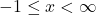## Convert [−1,∞) to inequality notation use the variable x.

Question

Convert
[−1,∞) to inequality notation use the variable x.

in progress 0
3 months 2021-07-31T17:33:38+00:00 1 Answers 11 views 0Step-by-step explanation:

We want to convert the interval [-1, ∞) to inequality notation using x as the variable.

The interval [-1, ∞) reads: “All real numbers between -1 and positive infinity.”

In other words, it is all numbers greater than or equal to -1 and less than positive infinity.

Therefore:Note that we do not use “or equal to” for the infinity as we can never really “equal” infinity.